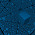Sunday, June 12, 2011

Kerala Engineering Entrance (KEAM) 2011 Questions on Kinematics in One Dimension

“Common sense is the collection of prejudices acquired by age eighteen.”

– Albert Einstein

Today we will discuss three questions (MCQ) on one dimensional kinematics. These questions were included in the KEAM (Engineering) question paper. They are of the type often seen in similar entrance tests. Here are the questions with their solution:

(1) A bus begins to move with an acceleration of 1 ms–2. A man who is 48 m behind the bus starts running at 10 ms–1 to catch the bus. The man will be able to catch the bus after

(a) 6 s

(b) 5 s

(c) 3 s

(d) 7 s

(e) 8 s

If the man can catch the bus after t seconds we have

10 t = 48 + ½ at2 where a is the acceleration of the bus (a = 1 ms–2).

[10 t is the distance covered by the man in time t and ½ at2 is the distance covered by the bus in the same time. Since the man is 48 m behind the bus, he has to cover an additional distance of 48 m].

Since a = 1 ms–2 the above equation reduces to

t2 – 20 t + 96 = 0

Therefore t = [–(–20) ±√{202 – (4×1×96}] /(2×1)

Or, t = [20 ±√16] /2 = 12 s or 8 s

The smaller time 8 s is the answer.

(2) A car moves a distance of 200m. It covers first half of the distance at speed 60 km h–1 and the second half at speed v. If the average speed is 40 km h–1, the value of v is

(a) 30 km h–1

(b) 13 km h–1

(c) 60 km h–1

(d) 40 km h–1

(e) 20 km h–1

The total distance moved is 200 m = 0.2 km

The total time (in hours) taken by the car is (0.1/60) + (0.1/v)

Since the average velocity is 40 km h–1, we have

40×[(0.1/60) + (0.1/v)] = 0.2

Or, 4/v = 0.2 – (4/60) = 8/60 from which v = 240/8 = 30 km h–1

[If v1 and v2 are the velocities while traversing the two halves of the path length s, the average velocity vav is given by

vav = s/[(s/2v1) + (s/2v2)]

Or vav = 2v1v2/( v1+v2)

Since this final equation contains velocities only you can make substitutions without confusion (no need of conversion of metre into kilometre when distances are to be handled).

Therefore, 40 = (2×60×v)/(60+v) from which v = 30 km h–1]

(3) A particle is moving with constant acceleration from A to B in a straight line AB. If u and v are the velocities at A and B respectively then its velocity at the mid point C will be

(a) [(u2 + v2)/2u]2

(b) (u + v)/2

(c) (v u)/2

(d) √[(u2 + v2)/2]

(e) √(v2 u2)/2

If ‘s’ is the length of the path AB, the velocity of the particle changes from u to v when it moves through the distance ‘s’. Therefore we have,

v2 u2 = 2as from which the acceleration, a = (v2 u2)/2s

If’ ’vc ’ is the velocity of the particle at the mid point C of the path AB, we have vc2 = u2 + 2a(s/2). Substituting for the acceleration ‘a’ from the above equation, vc = √[(u2 + v2)/2].

1 comment:

1.a very informative post.. from few days I was looking for such material as being enrolled in http://www.wiziq.com/course/2155-iit-jee-physics-video-tutorials-in-hindi I need such materials to boost up my preparation. Thanks :)